Courses

# Test: Final Accounts Of Manufacturing Entities - 1

## 29 Questions MCQ Test Principles and Practice of Accounting | Test: Final Accounts Of Manufacturing Entities - 1

Description
This mock test of Test: Final Accounts Of Manufacturing Entities - 1 for CA Foundation helps you for every CA Foundation entrance exam. This contains 29 Multiple Choice Questions for CA Foundation Test: Final Accounts Of Manufacturing Entities - 1 (mcq) to study with solutions a complete question bank. The solved questions answers in this Test: Final Accounts Of Manufacturing Entities - 1 quiz give you a good mix of easy questions and tough questions. CA Foundation students definitely take this Test: Final Accounts Of Manufacturing Entities - 1 exercise for a better result in the exam. You can find other Test: Final Accounts Of Manufacturing Entities - 1 extra questions, long questions & short questions for CA Foundation on EduRev as well by searching above.
QUESTION: 1

Solution:
QUESTION: 2

Solution:
QUESTION: 3

### Pick up the correct answer from the given choices(only one correct answer): Q.Goodwill is

Solution:
QUESTION: 4

Pick up the correct answer from the given choices(only one correct answer):

Q.Stock is

Solution:
QUESTION: 5

Pick up the correct answer from the given choices(only one correct answer):

Q.The manufacturing account is prepared:

Solution:
QUESTION: 6

A new firm commenced business on 1st January, 2006 and purchased goods costing Rs. 90,000 during the year. A sum of Rs. 6,000 was spent on freight inwards. At the end of the year the cost of goods still unsold was Rs. 12,000. Sales during the year Rs. 1,20,000. What is the gross profit earned by the firm?

Solution:
QUESTION: 7

From the following figures ascertain the gross profit: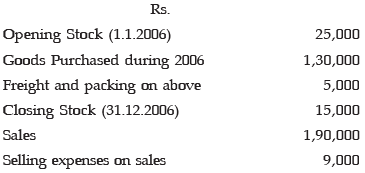Solution:

The correct option is B.

Gross profit = revenue sales- Cost of goods sold

Cost of goods sold= Opening Stock + Purchases + Direct expenses - Closing Stock

=25,000+1,30,000+5,000-15,000=₹1,45,000

Gross Profit=1,90,000-1,45,000=₹45,000

QUESTION: 8

A prepayment of insurance premium will appear in the Balance Sheet and in the Insurance Account respectively as:

Solution:

A current asset which indicates the cost of the premiums that have been paid in advance. It represents the amount that has been paid but has not yet expired as of the balance sheet date. In Insurance A/c in which it will be credited as it is an advance payment.

QUESTION: 9

Under-statement of closing work in progress in the period will

Solution:
QUESTION: 10

If sales revenues are Rs. 4,00,000; cost of goods sold is Rs. 3,10,000 and operating expenses are Rs.60,000, the gross profit is

Solution:
QUESTION: 11

Sales are equal to

Solution:
QUESTION: 12

A Company wishes to earn a 20% profit margin on selling price. Which of the following is the profit mark up on cost, which will achieve the required profit margin?

Solution:
QUESTION: 13

If sales are Rs. 2,000 and the rate of gross profit on cost of goods sold is 25%, then the cost of goods sold will be

Solution:

This can be represented as :
Let us assume the Cost Price is Rs.100
Profit Margin on Cost is @25% i.e. Rs.25
There fore the selling Price will be = Cost +Profit =SP
=Rs.100+Rs.25=Rs.125
If the Sales are Rs.2000
the cost of goods sold will be Rs.2000/125*100
Cost of good sole =Rs.1600.

QUESTION: 14

Sales for the year ended 31st March, 2005 amounted to Rs. 10,00,000. Sales included goods sold to Mr. A for Rs. 50,000 at a profit of 20% on cost.  Such goods are still lying in the godown at the buyer’s risk.  Therefore, such goods should be treated as part of

Solution:
QUESTION: 15

The capital of a sole trader would change as a result of:

Solution:
QUESTION: 16

Rent paid on 1 October, 2004 for the year to 30 September, 2005 was Rs. 1,200 andrent paid on 1 October, 2005 for the year to 30 September, 2006 was Rs. 1,600.  Rent payable, as shown in the profit and loss account for the year ended 31 December 2005, would be:

Solution:
QUESTION: 17

A decrease in the provision for doubtful debts would result in:

Solution:
QUESTION: 18

From the given information, choose the most appropriate answer: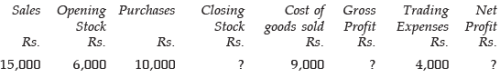Q.The value of closing stock is

Solution:
QUESTION: 19

From the given information, choose the most appropriate answer:Q.Gross profit will be

Solution:
QUESTION: 20

From the given information, choose the most appropriate answer:Q.Net profit will be

Solution:
QUESTION: 21

From the given information, choose the most appropriate answer: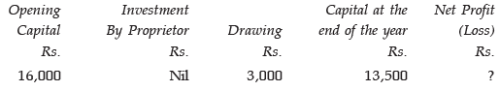Q.The net profit will be

Solution:
QUESTION: 22

From the given information, choose the most appropriate answer:Q.If in the given information, Net Loss is Rs. 1,000, then the investment made by the proprietor during the year will be

Solution:
QUESTION: 23

From the given information, choose the most appropriate answer: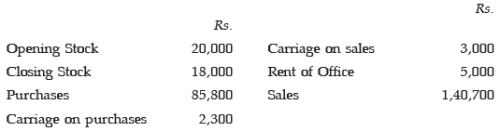Q.Gross profit will be

Solution:

The correct option is D.

Cost of goods sold=Opening stock+Purchases+direct expenses-Closing stock

=20000+85800+2300-18000

=90100

Gross profit=Revenue from sales- COGS

=140700-90100=₹50600

QUESTION: 24

From the given information, choose the most appropriate answer:Q.Net profit will be

Solution:
QUESTION: 25

The Zed Company, a whole seller estimates the following sales for the indicated months: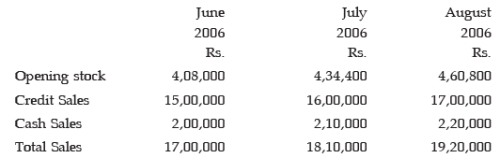Selling price is 125% of the purchase price.

Q.The cost of goods sold for the month of June, 2006 is:

Solution:
QUESTION: 26

The Zed Company, a whole seller estimates the following sales for the indicated months:Selling price is 125% of the purchase price.

Q.Stock purchased in July, 2006 is :

Solution:
QUESTION: 27

Considering the following information, answer the question given below: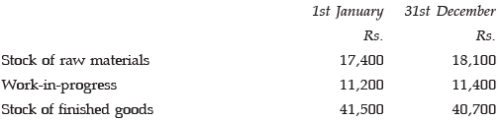During the year manufacturing overhead expenses amounted to Rs. 61,100, manufacturing wages to Rs. 40,400 and purchase of raw materials to Rs. 91,900.  There were no other direct expenses.

Q.The cost of raw materials consumed, issued and used were:

Solution:
QUESTION: 28

Considering the following information, answer the question given below:During the year manufacturing overhead expenses amounted to Rs. 61,100, manufacturing wages to Rs. 40,400 and purchase of raw materials to Rs. 91,900.  There were no other direct expenses.

Q.The manufacturing cost of finished goods produced were:

Solution:
QUESTION: 29

Considering the following information, answer the question given below:During the year manufacturing overhead expenses amounted to Rs. 61,100, manufacturing wages to Rs. 40,400 and purchase of raw materials to Rs. 91,900.  There were no other direct expenses.

Q.The manufacturing cost of finished goods sold was:

Solution: